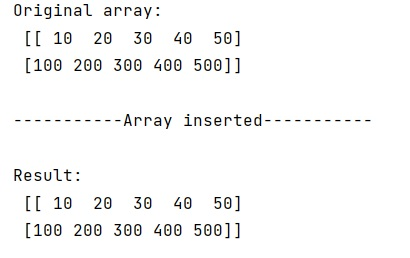# Concatenating empty array in NumPy

Learn, how to concatenate empty array in Python NumPy?
Submitted by Pranit Sharma, on February 07, 2023

NumPy is an abbreviated form of Numerical Python. It is used for different types of scientific operations in python. Numpy is a vast library in python which is used for almost every kind of scientific or mathematical operation. It is itself an array which is a collection of various methods and functions for processing the arrays.

## Concatenating an empty array in numpy

Suppose we are given a NumPy array that contains some numerical values and we need to concatenate an empty numpy array in this numpy array.

For this purpose, we will first create two NumPy arrays, one would be filled with values and the other array is the empty array. We will also define the data type for an empty array.

We will use the vstack() method to concatenate the empty array in the original numpy array.

The 'numpy.vstack() method is used to vertically stack an array to the existing array. This is equivalent to concatenation along the first axis after 1-D arrays of shape (N,) have been reshaped to (1, N).

Let us understand with the help of an example,

## Python code to concatenate an empty array in numpy

```# Import numpy
import numpy as np

# Creating a numpy array
arr = np.array([[10,20,30,40,50],[100,200,300,400,500]])

# Display original array
print("Original array:\n",arr,"\n")

# Creating an empty array
e_arr = np.array([], dtype=np.int64).reshape(0,5)

# Concatenating empty array to numpy array
res = np.vstack([arr, e_arr])

# Check that array is inserted
if res.any():
print("-----------Array inserted-----------\n")

# Display result
print("Result:\n",res)
```

Output: